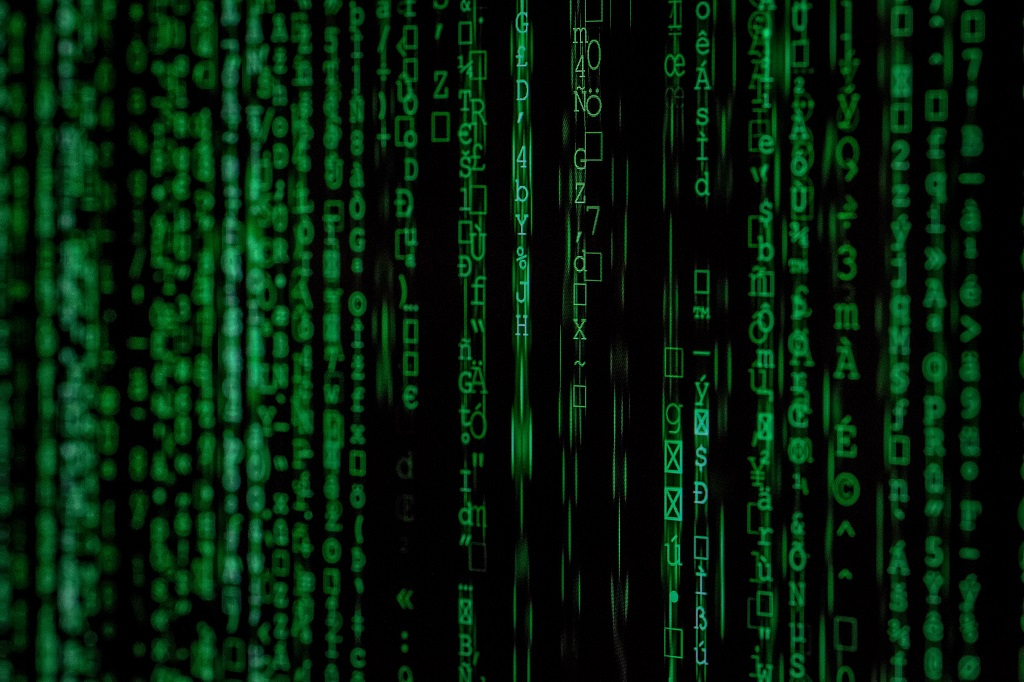# Understanding Binary Numbers

Yuk… Binary numbers. The subject of “binary numbers” has been the cause of arithmophobia (the irrational fear of numbers and arithmetic) in all systems and network admins. But without binary numbers computers and networking wouldn’t exist. All computers work in binary and also IP addressing works on the binary numbering system. An understanding of the binary system and the conversion of binary to decimal is essential for all anybody that is involved with computers and computer networking

Binary is something called a Base 2 numbering system so numbers will be a power of 2. All numbers can be broken down into 2 values, a 1 and a Zero. Binary numbers are very useful in electronics and computer systems. Digital electronics can easily work with a sort of “on” or “off” system where “on” is a 1 and “off” is a zero. Often times the 1 is a “high” voltage, while the 0 is a “low” voltage or ground.

### How do binary numbers work?

Binary numbers only use the numbers 1 and 0. In a binary number each “place” represents a power of 2. For example:

1 = 20 = 1
10 = 21 = 2
100 = 22 = 4
1000 = 23 = 8
10000 = 24 = 16

### Converting from Binary to Decimal

If you want to convert a number from binary to decimal, you can add up the “places” that we showed above. Each place that has a “1” represents a power of 2, starting with the 0s place.

Examples:

101 binary = 4 + 0 + 1 = 5 decimal
11110 binary = 16 + 8 + 4 + 2 + 0 = 30 decimal
10001 binary = 16 + 0 + 0 + 0 + 1 = 17 decimal

Another example of Binary Calculation-

The below binary number of 11001000 can be explained easily using the below table.